# On the cover: Regular tilings

Poppy Azmi explores the patterns that are all around us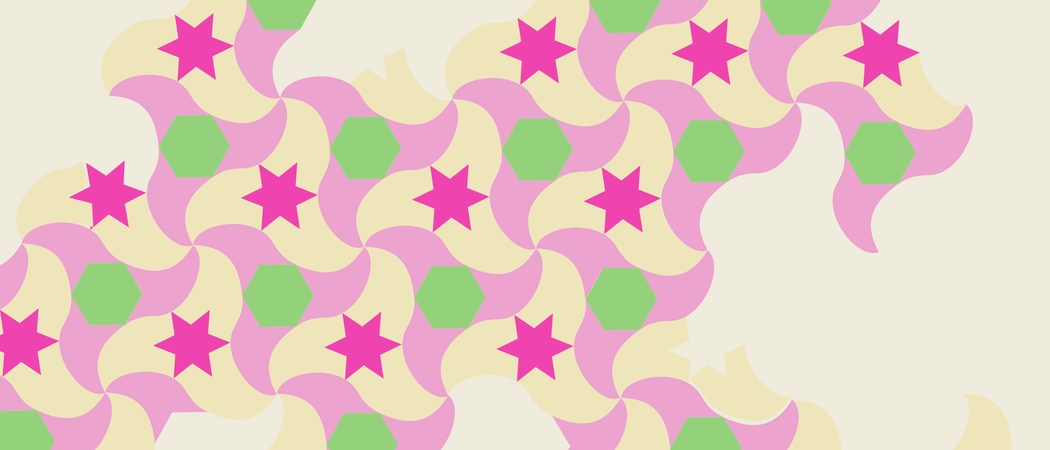Tilings have been studied for thousands of years dating back to 3000BC, and people are still finding out more and more about them now. They’ve been used in architecture for longer, and appear all over the natural world. I’m going to try and present a small subtopic within tilings that will hopefully be interesting and straightforward to understand.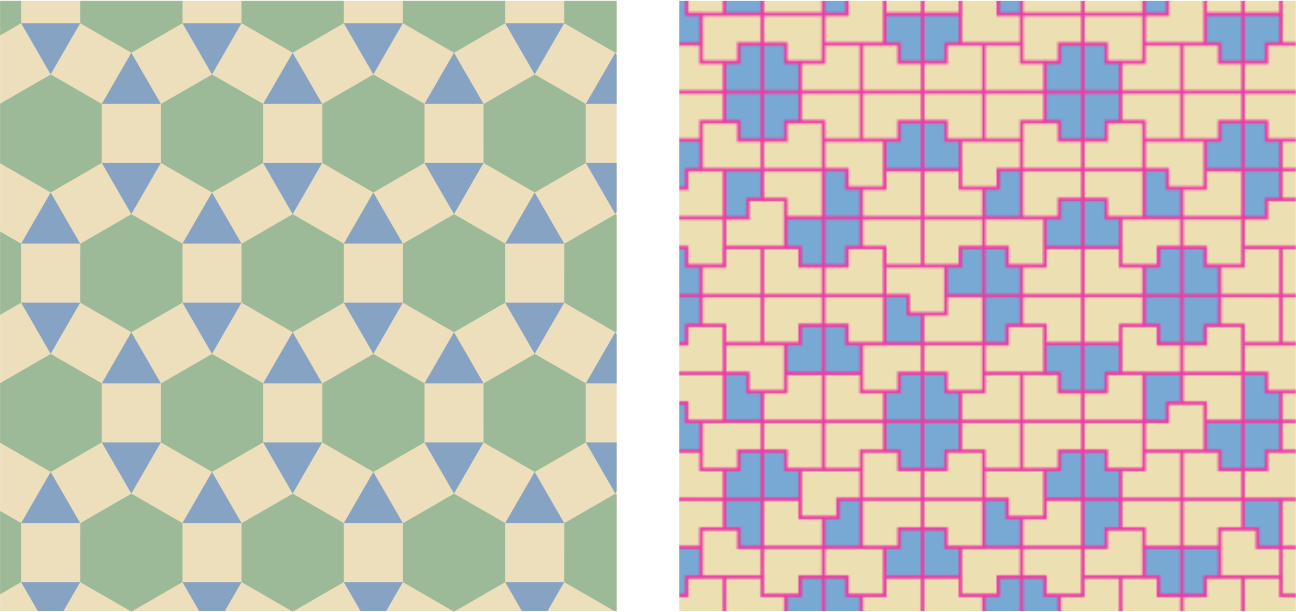The rhombitrihexagonal tiling from around 200 BC (left) and the Ammann chair tiling from AD 1977 (right). Mathematica code by Sasho Kalajdzievski, CC BY-NC-SA 3.0

## Second to the right and tile on till morning

A tiling problem, in the context of this topic, is the following: given a set of tiles (closed simple curves and their interior) can we completely cover the plane without gaps or overlaps?

The tilings which are easiest to visualise and draw are tilings by regular polygons, which I will call regular tilings here for simplicity. A lot of the results I’m going to mention can be extended to non-regular polygons, but for now let’s stick to maths which is a bit easier to deal with.

Let’s even start with the simplest kind of regular tiling: tilings that are monohedral, which means only one type of tile is used to construct it.

So how can we construct a monohedral regular tiling? Let’s start with the most common: a tiling of the plane by squares. It is easy to see how it is constructed and why it tiles the plane, simply because a square has four corners with four right angles. We know it is possible to place four squares together, edge to edge, so that all four of them have a common corner, simply because $4 \times \pi/2 = 2\pi$, which is the number of radians around a point. We can do this indefinitely so that each corner in the tiling is a corner common to four squares:A slightly more complicated tile is the regular hexagon. See if you can work out how to prove that regular hexagons tile the plane.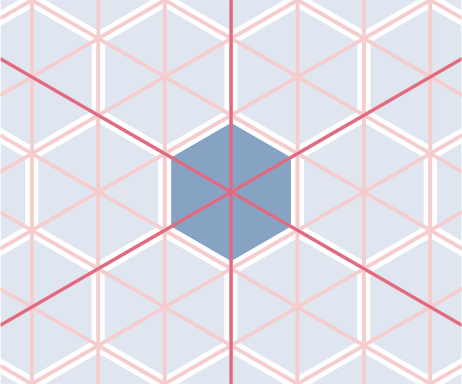There is only one more monohedral regular tiling of the plane, and it can be constructed using the hexagon tiling. Take a regular hexagon and draw in its three axes of reflectional symmetry. This gives us a regular tiling with only equilateral triangles. These are actually the only three monohedral regular tilings of the plane! I will prove this later on.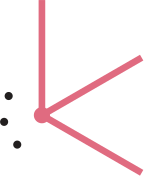Now I want to discuss how to construct a vertex (ie a point where at least three tiles meet) in a regular tiling. We know there are $2\pi$ radians around any vertex. Because we’re working with regular polygons, there’s a smaller number of angles that we have to choose from to make up this $2\pi$. Could we cut this down concretely?

It is a well known fact that the angle sum in a convex $n$-polygon, a polygon with $n$-sides, is $(n-2)\pi$ (to prove this, consider splitting polygons up into triangles!). From now on, when I talk about polygons I mean regular polygons.

## Counting corners

If we consider a tiling where each vertex has $r$ polygons around it, polygons with $n_1$, $n_2$, … up to $n_r$ sides respectively, then these two facts combined give us the equation $2\pi=\frac{(n_1-2)\pi}{n_1}+\cdots+\frac{(n_r-2)\pi}{n_r}.$ By cancelling $\pi$ on both sides, we get \begin{equation}\label{eq:anglesum} \frac{n_1-2}{n_1}+\cdots+\frac{n_r-2}{n_r}=2. \tag{*} \end{equation}

So any polygons around a vertex in a regular tiling have to satisfy this equation. I think this is quite cool, because now we’ve got a concrete equation that we can work with algebraically!

This equation can be used to prove there are only three monohedral regular tilings of the plane. Monohedral means that all $n_i = n$, for some integer $n$ at least 3. Then \eqref{eq:anglesum} simplifies to $\frac{n-2}{n}+\cdots+\frac{n-2}{n}=2\quad\Rightarrow\quad r\frac{n-2}{n}=2.$

Writing this in terms of $n$ gives $n=2+\frac{4}{r-2}.$ We know that $n$ is an integer, which means that $r-2$ must divide 4. This implies $r – 2 = 1, 2$, or $4$, and so $r = 3, 4,$ or $6$. This is equivalent to saying, the only monohedral regular tilings of the plane are by triangles, squares, and hexagons!

## A vertex by any other name would look the same

One property about monohedral regular tilings is that all of the vertices ‘look’ the same. This leads onto the question: what about other regular tilings where all the vertices look the same? Equation \eqref{eq:anglesum} seems like it could be quite helpful with figuring out how many different regular tilings we can form this way.

I’m going to cheat a little with this part, and just tell you that there are exactly 21 ways to satisfy \eqref{eq:anglesum}. It is also noteworthy that the order of the $n_i$’s does matter in this case because reading off the polygons around a vertex in a different order makes a different tiling. I’ve not derived or included a proof of this result because it is quite long and tedious.

There is actually a problem here. If for example we take the numbers 3, 4, 4, and 6 for the $n_i$’s. These fit the equation, but we can’t extend this arrangement of regular polygons around a vertex to a full tiling of the plane. This is because as shown below, at the black vertex we are forced to place the polygons in the order triangle-square-hexagon-square, but this is not in the order 3, 4, 4, 6. So not all vertices have the same arrangement of polygons, and this doesn’t extend to a tiling with the property we want.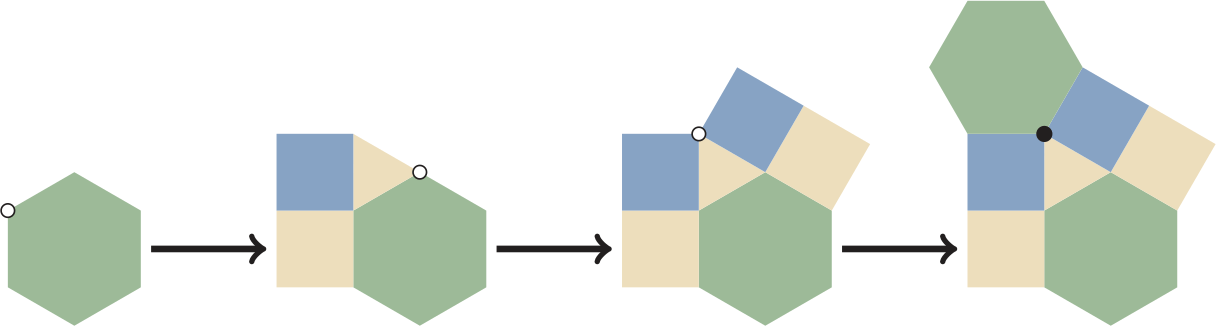Trying to build the 3, 4, 4, 6 tiling by adding polygons around each vertex (indicated with a white dot) in the order triangle-square-square-hexagon.

Just because an arrangement of polygons fits around a vertex edge-to-edge, doesn’t mean this extends to a tiling of the plane where all vertices are the same type. Have a think about why this is. For this reason, it turns out there are only 11 ways to tile the plane in the way we want to.

Showing this requires two steps. I’m not going to be super meticulous here and do this for all 21 different options, but I’ll do an example for each step.

1. We need to show that for 10 types of vertices, we can’t extend from the region around the starting vertex to a tiling of the plane, like we did for the numbers 3, 4, 4, 6 above.
2. We need to show that the remaining 11 types of vertices actually lead to tilings of the plane. We already showed this for the monohedral tilings, and in the picture below I show it for constructing a tiling with polygons 3, 6, 3, 6 from the monohedral triangle tiling:Constructing tiling with polygons 3, 6, 3, 6 starting with the monohedral tiling by equilateral triangles. Shaded regions are where the hexagons come from.

These 11 tilings are known as the Archimedean tilings. So now we’ve shown that there are only 11 regular tilings where each vertex has the same arrangement of polygons around it! This, to me, is a good example of why maths is so interesting. We started with a problem that appeared to have an infinite number of solutions and managed to use only basic high school techniques and knowledge to deduce that there were only 11 different solutions instead!

## Beyond Archimedean tilings

The Archimedean tilings all have another property, and that is that they are periodic, invariant under translations in two non-collinear directions. I find periodic tilings really interesting as it’s fairly easy to see how they’re constructed.

I also study aperiodic tilings, tilings that have no translational invariance. These can be studied using other branches of maths, such as topology. There is an object called the tiling space which is defined as all the translations of a tiling. For the grid unit square tiling, the tiling space is a torus. This is because the tiling is equivalent under translation by one tile horizontally or vertically, so opposite edges of a square are identified, which gives a flat torus.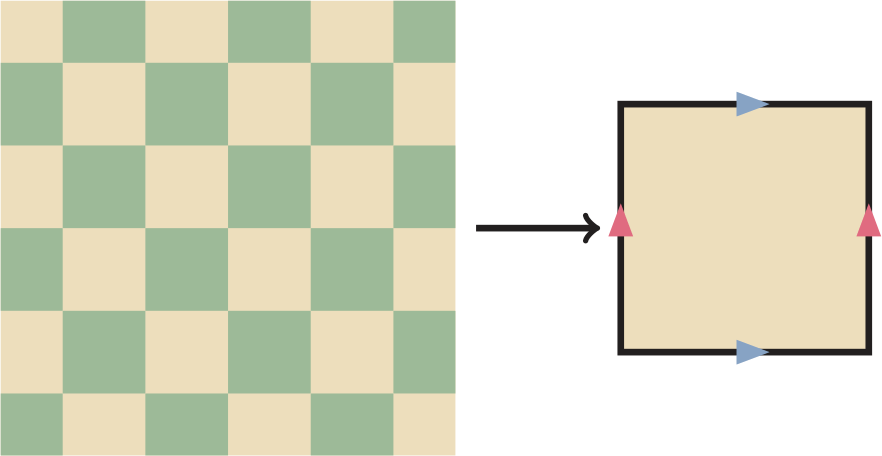The tiling space of every periodic tiling is always the torus, so this topological approach isn’t so helpful for periodic tilings, but it is for aperiodic tilings. If you’re interested to learn more, a good article to introduce the subject is A primer of substitution tilings on the Euclidean plane by Natalie Frank. And, if you’re interested in geometry and tilings, then consider reading the book Tilings and patterns by Branko Grünbaum and Geoffrey Colin Shephard. You could also look into Platonic solids, which are an introduction to some of these ideas in three dimensions.Poppy is currently completing her master’s at Durham University. This article is based on material she learned during her final year project. In general she’s interested in geometry, algebra and topology.